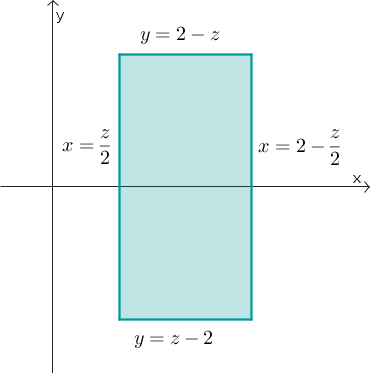# Math Insight

### Image: Triple integral rectangular cross sectionThe rectangular region \begin{gather*} \frac{z}{2} \le x \le 2-\frac{z}{2}\\ z-2 \le y \le 2-z \end{gather*} was calculated as a cross section perpendicular to the $z$-axis of the three-dimensional region bounded by the planes $z=0$, $z=4-2x$, $z=2-y$, $z=2x$, and $z=2+y$.

Image file: triple_integral_rectangle_cross_section.png

Source image file: triple_integral_rectangle_cross_section.ggb
Source image type: Geogebra